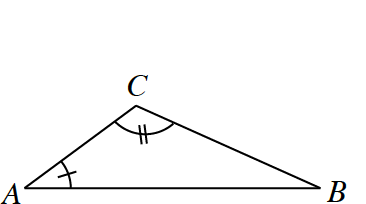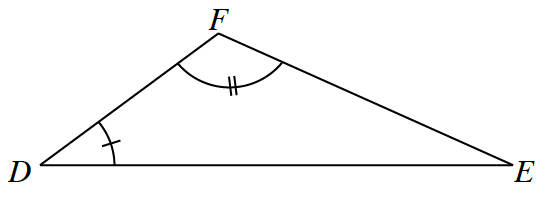### Home > INT2 > Chapter 2 > Lesson 2.3.1 > Problem2-85

2-85.

One possible proportion for the similar triangles below is $\frac { A C } { A B } = \frac { D F } { D E }$. Write at least three more proportions given that $∆ABC\sim ∆DEF$.Using this proportion, think of at least two more proportions to satisfy the question.

$\frac{BC}{EF}= \frac{AB}{DE}$